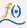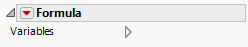Publication date: 11/29/2021

## Formula

The Formula elementshows the graphs of a function, its inverse, or a parametric curve. The function or functions are defined by column formulas.

y = f(x):

To plot a function of a single argument across the values of its single argument, drag the column that contains the formula to the Y zone and the column that contains the values of its single argument to the X zone.

Make sure that the Response Axis is set to Auto or Y.

y = f -1(x):

To plot the inverse of a function of a single argument for a column of values, drag the column that contains the formula to the X zone. Drag the column that contains the values of interest to the Y zone.

Set the Response Axis to X.

x = f(t) and y = g(t): To plot the parametric curve defined by two functions, drag one to the X zone and the other to the Y zone.

Note: Because the Formula element plots the values of a function, the plot is not affected in any way if rows are hidden and not excluded.

Figure 3.40 Formula OptionVariables

Shows or hides graph elements for variables, or re-orders the display of variables.

Note: These options do not apply to variables in the Group X, Group Y, Wrap, or Page zones.

Check boxes are followed by the zone designation and the name of the variable. Use check boxes to do the following:

Show or hide the elements corresponding to a variable in a zone.

Add or remove the effect of applying the Color, Size, Shape, or Freq variable to the variable in the zone.

Tip: If you have multiple graphs, you can color or size each graph by different variables. Drag a second variable to the Color or Size zone, and drop it in a corner. In the Variables option, select the specific color or size variable to apply to each graph.

Use arrows to re-order the display if there are multiple variables in a zone. Highlight a variable name and click an arrow to reposition it.

For an example using Variables, see Example of an Area and Line Chart.

#### Red Triangle Option for Formula

Response Axis

Specifies the axis for the formula variable.